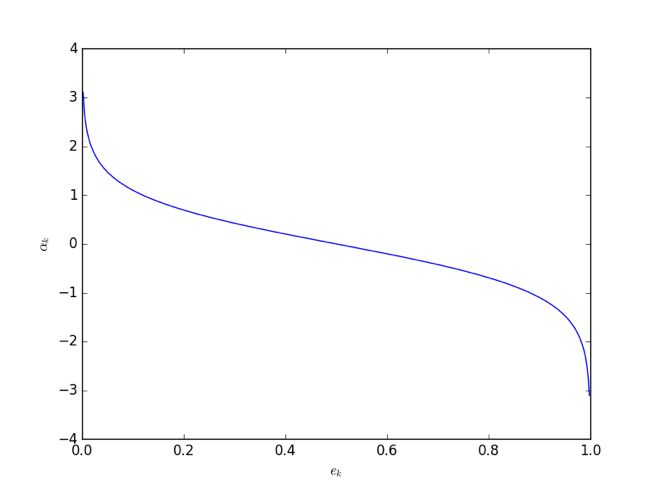• 如何根据弱模型的表现更新训练集的权重
• 如何根据弱模型的表现决定弱模型的话语权

1. 输入：训练数据集（包含 N 个数据）、弱学习算法及对应的弱分类器、迭代次数 M
2. 过程
1. 初始化训练数据的权值分布
2. $k = 0,1,\ldots,\ M - 1$
1. 使用权值分布为$W_{k}$的训练数据集训练弱分类器
2. 计算$g_{k + 1}(x)$在训练数据集上的加权错误率
3. 根据加权错误率计算$g_{k + 1}(x)$的“话语权”
4. 根据$g_{k + 1}(x)$的表现更新训练数据集的权值分布：被$g_{k + 1}\left( x \right)$误分的样本（$y_{i}g_{k + 1}\left( x_{i} \right) < 0$的样本）要相对地（以$e^{\alpha_{k + 1}}$为比例地）增大其权重，反之则要（以$e^{- \alpha_{k + 1}}$为比例地）减少其权重 这里的$Z_{k}$是规范化因子 它的作用是将$W_{k + 1}$归一化成为一个概率分布
3. 加权集成弱分类器
3. 输出：最终分类器$g(x)$